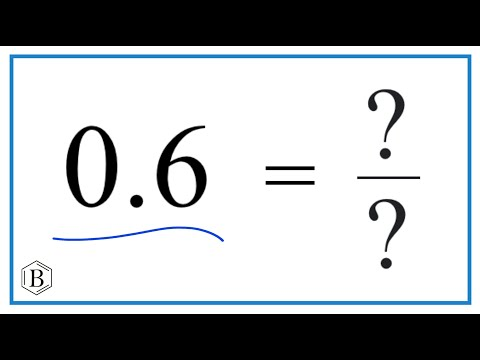Home » What is 0.6 as a Fraction? – Decimal to Fraction Conversion

# What is 0.6 as a Fraction? – Decimal to Fraction Conversion

What is 0.6 as a Fraction?

• A. 17/34
• B. 7/20
• C. 1/2
• D. 3/5
• E. 129/100

## Solution:

0.6 can be expressed as the fraction 3/5.

Here’s a quick explanation:

The decimal 0.6 is in the tenths place, which means it can be expressed as a fraction greater than 10. So we’ll start with a 6/10.

To simplify, take the greatest common divisor (GCD) of 6 and 10, which is 2. Divide the numerator (6) and denominator (10) by 2 to get 3/5.

##### Explanation

Converting decimals to fractions may appear difficult at first, but with the right approach, it becomes simple. The process involves determining the decimal’s place value and then simplifying the fraction. Let’s break these steps down as we convert 0.6 to a fraction.

## Understanding the Decimal Place

Understanding the decimal place value is the first step in converting a decimal to a fraction. In this case, the decimal number is 0.6. The ‘6’ in this case is in the tenths place. This is because the first digit to the right of the decimal point represents tenths in the decimal number system. As a first step, we can express the decimal 0.6 as the fraction 6/10.

## Simplifying the Fraction

The next step is to make this fraction as easy as possible. When the greatest common divisor (GCD) of the numerator and the denominator is 1, the fraction is in its simplest form. This means that the only thing the numerator and denominator have in common is 1. In the case of 6/10, the largest number that both 6 and 10 can be divided by is 2.

## 0.6 as a Fraction in Simplest Form

We divide the numerator (6) and denominator (10) by their respective GCDs, which is 2, to simplify the fraction 6/10. This results in a result of 3/5 (since 6 ÷ 2 = 3 and 10 ÷ 2 = 5). Therefore, the simplest fractional representation of the decimal 0.6 is 3/5. This technique can reduce any decimal to its most basic fractional form.

In conclusion, to convert a decimal to a fraction, one must first comprehend decimal place values before expressing the decimal as a fraction and, if necessary, simplifying the fraction.